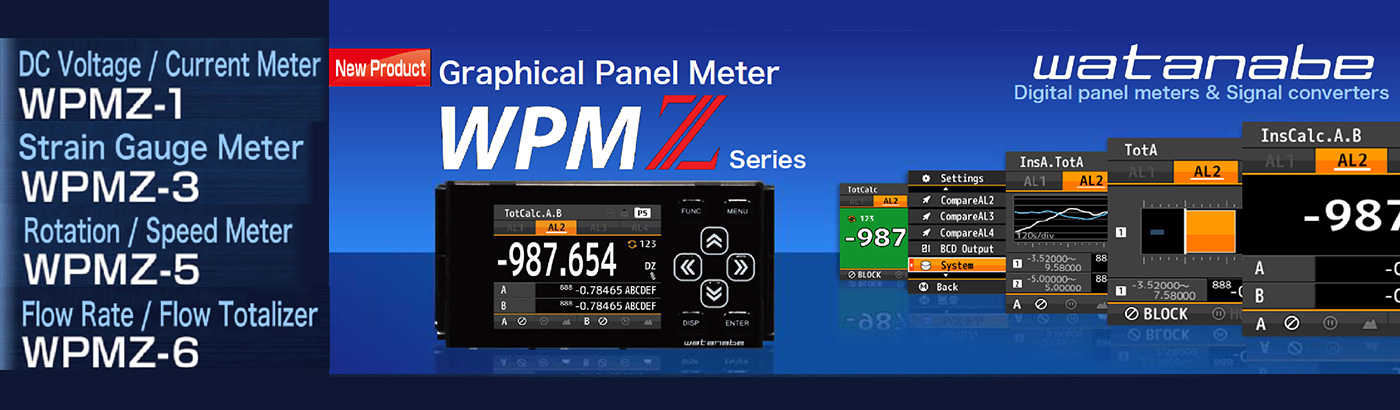Rexis Sdn Bhd
Lot 2-2-38, 2nd Floor,
Wisma Rampai,
Jalan 34/26,
Taman Sri Rampai,
53300 Kuala Lumpur,
Malaysia.
+603-4149 9398
+603-4141 6385

## WPMZ-1 Thickness measurement by 2ch input

08 Sep 2021View Full Size
Calculation result of 2 channel input =Thickness value

Example of Calculation method

C =x-y
x=Total length y=Rated width

(A+B)+C) x K = Thickness value
A = Sensor A value (Ach input) B = Sensor B value (Bch input)
C = Total length (between A & B) – Rated width K = -1
*C & K can set value by setting.

For example, if you want to display zero at 2cm width,
and total length between A & B is 10cm, set 8 as C by setting. Then calculation result will be difference from 2cm rated width.